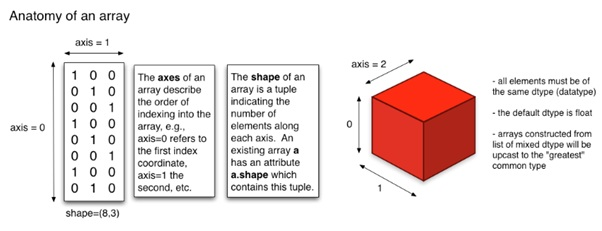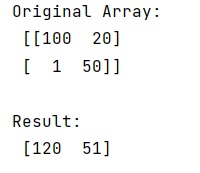# How does the axis parameter from NumPy work?

Learn, how does the axis parameter from NumPy work in Python?
Submitted by Pranit Sharma, on February 20, 2023

NumPy is an abbreviated form of Numerical Python. It is used for different types of scientific operations in python. Numpy is a vast library in python which is used for almost every kind of scientific or mathematical operation. It is itself an array which is a collection of various methods and functions for processing the arrays.

## Understating the working of axis parameter

We can understand the concept of axis with the following image representation:The shape of the (boolean) array in the above figure is shape=(8, 3). The ndarray.shape() will return a tuple where the entries correspond to the length of the particular dimension. In our example, 8 corresponds to the length of axis 0 whereas 3 corresponds to the length of axis 1.

In other words, when we need to operate some function on a row, we use axis=1 and in the case of a column, we use axis=0.

Let us understand with the help of an example,

## Python code to demonstrate how does the axis parameter from NumPy work

```# Import numpy
import numpy as np

# Creating a numpy array
arr = np.array([[100,20],[1,50]])

# Display original array
print("Original Array:\n",arr,"\n")

# Finding sum of array along the row
res = np.sum(arr,axis=1)

# Display Result
print("Result:\n",res)
```

Output: